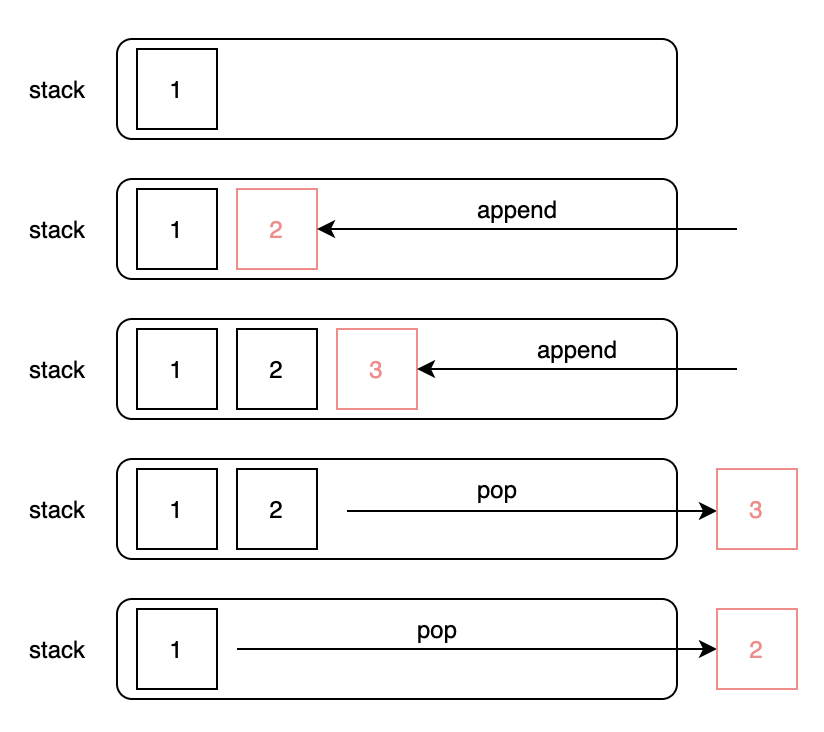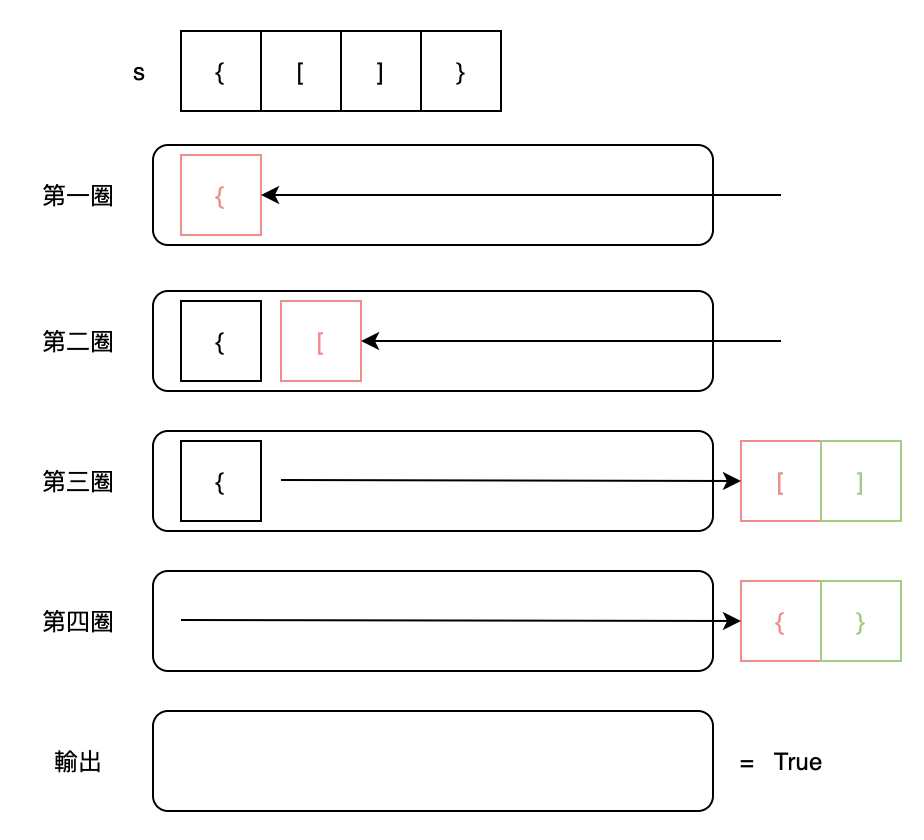#DAY 5
1
Software Development

## 簡單說說 Stack

Stack（堆疊）是一種後進先出 ( LIFO ) 的資料結構，新增資料永遠從後面進去，取出資料永遠從最後面資料取出，使用的方式及過程如下圖。## 題目解說

• 1 <= s.length <= 104
• 字串是由'() [] {}'組成的

``````輸入: s = "()"

``````

``````輸入: s = "()[]{}"

``````

``````輸入: s = "(]"

``````

``````輸入: s = "([)]"

``````

``````輸入: s = "{[]}"

``````

## 解題的想像

### 文字描述

1. 宣告一個stack
2. 跑一個迴圈每一圈檢查一個字元
• 遇到一個開括號就放進stack中
• 遇到一個關括號就從stack取出一筆資料檢查是否有配對成功
• 若沒有配對成功直接回傳False結束
• 若有配對成功繼續往下個字元檢查
3. 最終如果stack是空的，代表都有配對到是平衡的，回傳True

### 圖解過程## 程式碼撰寫

``````class Solution:
def isValid(self, s: str) -> bool:
stack = []
for c in s:
# 遇到開括號，將值放入stack中。
if c == '{' or c == '[' or c == '(':
stack.append(c)
# 遇到關括號，跟stack確認是否有配對到。
else:
if not stack: return False
open = stack.pop()
if c == '}' and open == '{': continue
elif c == ')' and open == '(': continue
elif c == ']' and open == '[': continue
else: return False
return not stack
``````

## 希望您今天能瞭解到...

1. Stack基礎概念
2. 20. Valid Parentheses 解題方法

Next：232. Implement Queue using Stacks